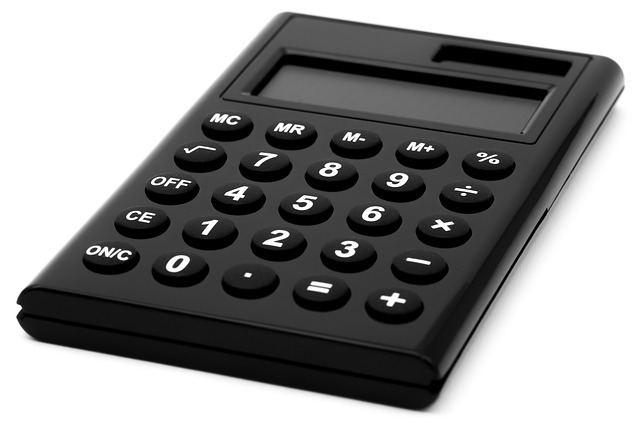### ReviewEcon.com

AP, IB, and College Microeconomic and Macroeconomic Principles

# 9 Microeconomics ​Formulas to Review Before the Exam

Updated 4/13/2021 Jacob Reed
Below you will find all the formulas you need to know for microeconomics. The microeconomics exam doesn’t generally have quite as much math as the macroeconomics exam so the list below isn’t overly long, but make sure you review it all before exam day! If you want some examples and explanations, watch the youtube video.Total Revenue Test for determining price elasticity of demand.
P x Q = Total Revenue
If higher prices means higher total revenue, the price elasticity < 1 or Inelastic price elasticity of demand
If higher prices means lower total revenue, the price elasticity > 1 or Elastic price elasticity of demand

Elasticity Coeffieicents
% Change Quantity/%Change in Price (for price elasticity)
Absolute value > 1 means elastic
Absolute value < 1 means inelastic
Cross-price elasticity: Negative = Complement; Positive = Substitute
Income elasticity: Negative = Inferior Good; Positive = Superior or Normal Good

Profit Maximizing point: MC=MR Firms should continue to produce more output until they reach this point. Until then MC<MR

Cost-Benefit: You should continue doing something until the MC=MB

Utility per dollar maximization: if the marginal utility per dollar for good A is greater than the utility per dollar for good B, you should consume less of good B and more of good A until MUA/PA=MUB/PB

Lowest Cost Resource Combination of production: You should use more of the resource with a greater Marginal Revenue
Product per dollar and less of the resource of with less Marginal Revenue Product per dollar until MPL/PL=MPC/PC

Marginal Cost of labor: MC=WL/MPL

Marginal Revenue Product: Δ Total Revenue/Δ Quantity of resource OR Marginal Product x Price